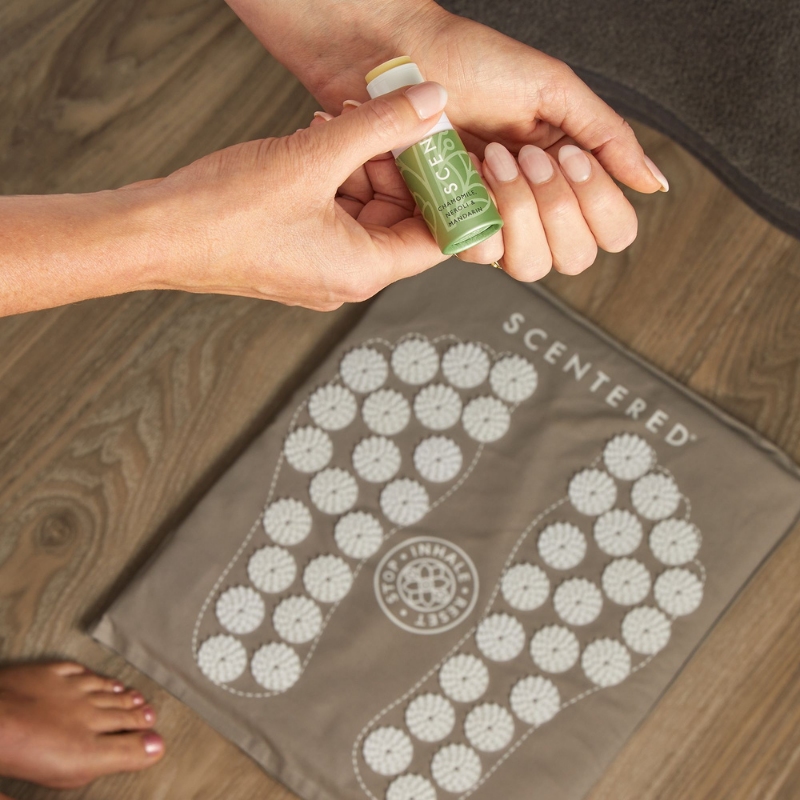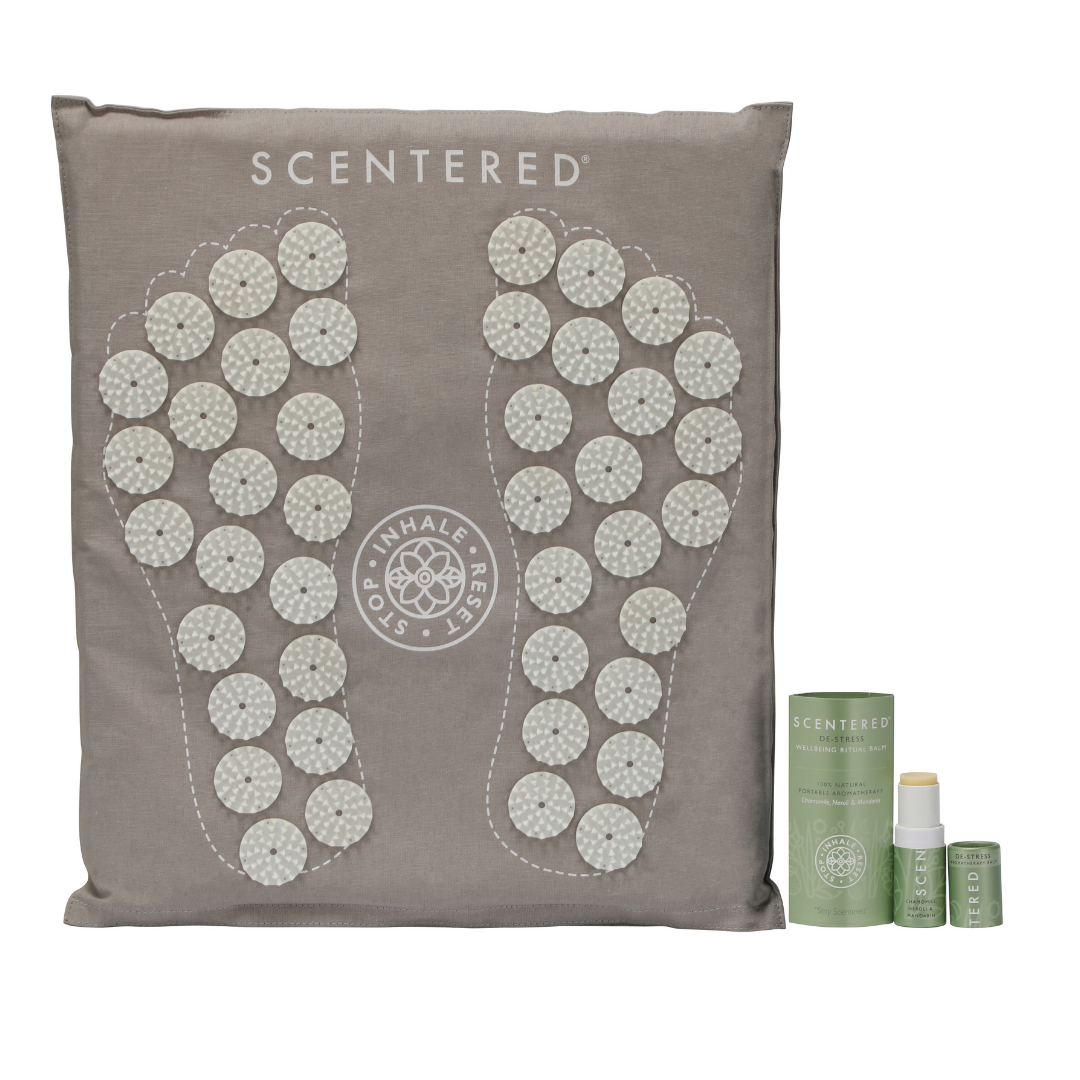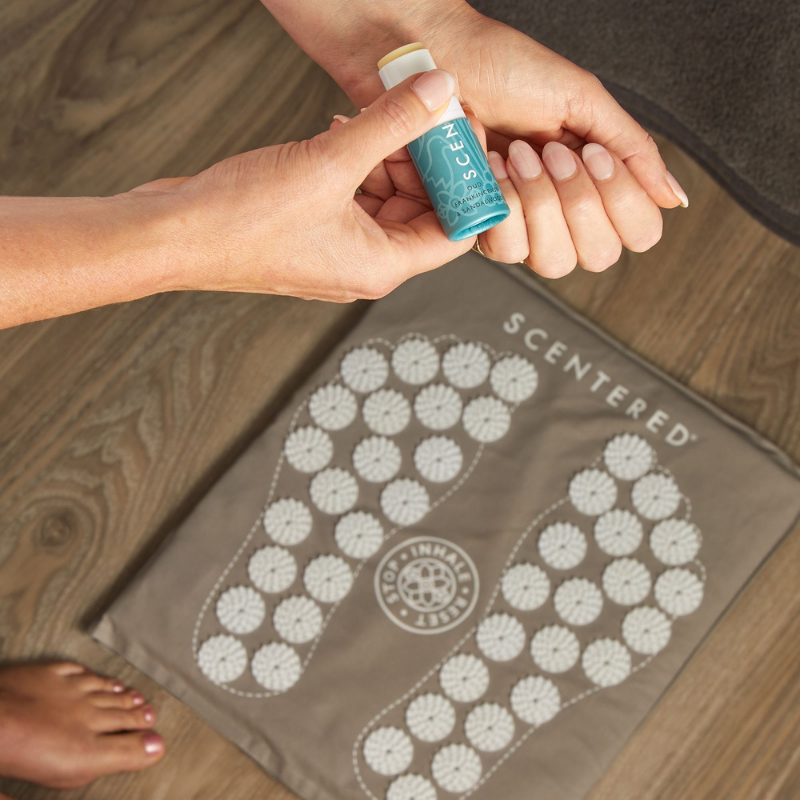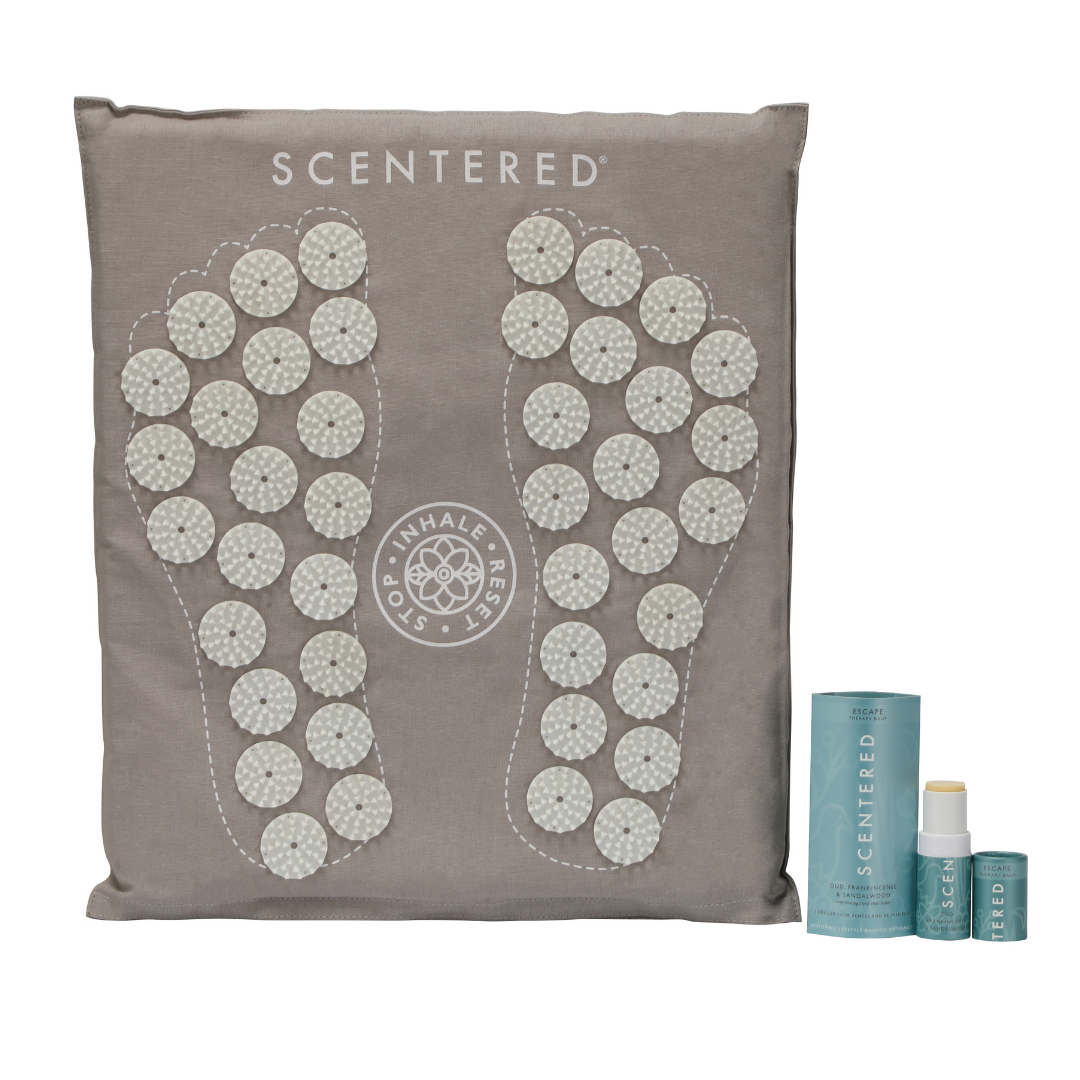# Aromatherapy Balms### S\$L\$E\$E\$P\$ W\$E\$L\$L\$ W\$e\$l\$l\$b\$e\$i\$n\$g\$ R\$i\$t\$

De £16.00### D\$E\$-S\$T\$R\$E\$S\$S\$ W\$e\$l\$l\$b\$e\$i\$n\$g\$ R\$i\$t\$u

De £16.00### De-stress Five Minute Foot Mat & Mindful Aromatherapy Balm

£35.00### S\$i\$g\$n\$a\$t\$u\$r\$e\$ R\$i\$t\$u\$

£69.00### H\$A\$P\$P\$Y\$ W\$e\$l\$l\$b\$e\$i\$n\$g\$ R\$i\$t\$u\$a\$

De £16.90### L\$O\$V\$E\$ W\$e\$l\$l\$b\$e\$i\$n\$g\$ R\$i\$t\$u\$a\$l

De £16.90### E\$S\$C\$A\$P\$E\$ W\$e\$l\$l\$b\$e\$i\$n\$g\$ R\$i\$t\$u\$a

De £16.90### F\$O\$C\$U\$S\$ W\$e\$l\$l\$b\$e\$i\$n\$g\$ R\$i\$t\$u\$a\$

De £16.90### D\$a\$i\$l\$y\$ R\$i\$t\$u\$a\$l\$

£38.90### B\$e\$ K\$i\$n\$d\$ T\$o\$ Y\$o\$

£39.00### Escape Five Minute Foot Mat & Mindful Aromatherapy Balm

£35.00### Sleep Well & De Stress Aromatherapy Balm Duo

£27.00### Happy and Sleep Well Aromatherapy Balm Duo

£27.00£29.00